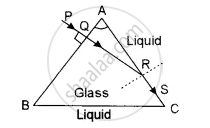# A Narrow Beam of Monochromatic Light, Pq, is Incident Normally on One Face of an Equiangular Glass Prism of Refractive Index 1.45. When Th - Physics (Theory)

Numerical

A narrow beam of monochromatic light, PQ, is incident normally on one face of an equiangular glass prism of refractive index 1.45. When the prism is immersed in a certain liquid, the ray makes a grazing emergence along the other face (See figure). Find the refractive index of this liquid.#### Solution

When the prism is immersed in the liquid and the incident ray emerges along AC, it is clear that it must be incident at the critical angle C on the face AC.
∠A + ∠ARQ = ∠C + ∠ARQ

From the figure, ∴ ∠C = ∠A = 60°
The critical angle when the prism is immersed in the liquid is 60°.
If μg is the refractive index of the material of the prism w.r.t. liquid

Then ""^l µ_g = 1/sin"C" = 1/sin60° = 1/(sqrt3/2) = 2/sqrt3

""^l µ_g = 2/sqrt3

Also, we know that ""^l µ_g =( ""^aµ_g)/( ""^aµ_l)

""^aµ_l =( ""^aµ_g)/( ""^l µ_g )

= 1.45/2 xx sqrt3

= 1.45 x 0.866

= 1.256

Concept: Dispersion by a Prism
Is there an error in this question or solution?